Contents >> Engineering Mathematics >> Control Systems >> Dynamic Analysis of Control System of Hydraulic Drive >> Algorithms of forming and solving of mathematical model

 Automatic control systems - Algorithms of forming and solving of mathematical model Algorithms of forming and solving of mathematical model Forming of initial data. All initial information for executing of the dynamic analysis consists of two parts: - information on structure of the considered circuit; - information about physical and design data of elements of the circuit. The first part of the initial information is designed according to the method of structural description of the hydraulic circuits stated above, based on classification of base hydraulic elements, introduction of the generalized linear dynamic LINK with nodes i (input), j (output) and k (an additional input for a link – the summer). On the basis of it each dynamic link has received the identifier е (LINK) and the code unequivocally defining group of the equations for the mathematical description of the certain type link. According to it the special table is developed for the task of the initial data of dynamic links, having the following structure:(6) where n – number of a link; А – matrix-row of the factors describing physical, geometrical and design data of the link that makes the contents of the second part of the initial information. At the majority of the links having only two nodes, the third node is designated by zero. Number of a link n is intended for a choice of the necessary file of factors from the general field of initial data at forming of the general system of the equations. Forming of mathematical model of a control system. As a result of the analysis of a line of the table of input data (6) for each dynamic link depending on its type the system of the differential and-or algebraic equations is designed as follows: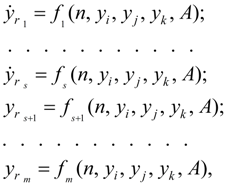(7) where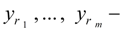are phase variables in nodes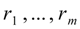; т – dimension of system of equations of  the given type link; s – dimension of system of differential equations;accept values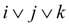, i.e. numbers of the nodes corresponding to the given link; the concrete kind of the right parts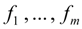, physical sense of variables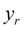, dimensions m and s are unequivocally defined by the type of the link. The description of each link of dynamic system is realized by two separate blocks: D (the differential equations of mathematical model of the link) and-or A (the algebraic equations of mathematical model of the link). On the basis of the initial information the following fields of initial data are formed. Matrix of communications:(8) differing matrix S [see (1)] presence of an additional column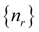of numbers of links. Matrix of parameters of links: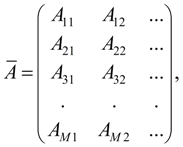(9) where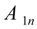– arrays of parameters of the 1-st type links;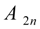– arrays of parameters of the 2-nd type links and so on;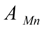– arrays of parameters of the M type links; М – number of types of links in the considered classification (can be replenished); n =1, 2, … – numbers of the given type links. Forming of mathematical model of a control system is executed as follows. The link( т =1, ... , N ) is considered, comparison of  its type with each of possible is made. At concurrence to one of them the reference to corresponding this type of link to the block differential ( D ) and-or algebraic ( A ) equations with transfer of the specification:is made. By means ofelement(a row of factors) gets out from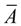, where I corresponds to the type of link defined before this. As a result the required equations in which the necessary factors are substituted get out of the general library of mathematical models. Then there is a transition to a following link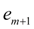, etc. until the list of elements of system (a column е of matrix) will be completely settled, that means the process of forming of system of the equations has been completed. External influences and control signals. The model control (solution of system of the equations) is executed by the special block which function includes generating signals of external influence. In the control block it is possible to simulate as typical external influences (sine wave, spasmodic, linear, etc.), and non-standard, taking place in most cases and defined by a specific problem. Moreover, for consideration of problems with changing physical and design data in the control block it is possible to provide the task of these variables as function of time or other variable received as a result of the solution. Besides it is obviously possible to task in the control block operating influences and forces of resistance as the functions approximated by a final set of discrete values or having a certain spectrum. Algorithm of solving. For integration of systems of the differential equations now there is a huge quantity of methods of numerical integration (Runge-Kutta method, Euler’s method and its updatings, Gere's method, etc.) on the basis of which the set of various programs is developed, that allows always to choose the suitable instrument for the problem decision.

 < Previous Contents Next >